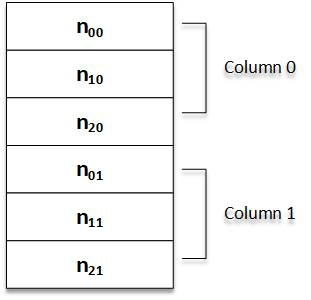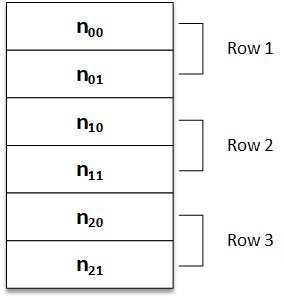# Array C Programing

Let a be a two dimensional m x n array. Though a is pictured as a rectangular pattern with m rows and n columns, it is represented in memory by a block of m*n sequential memory locations.

However the sequence can be stored in two different ways:

1. Column Major Order
2. Row Major Order

In the column major order, the elements are stored column by column. First column, second column and so on

For int nuber; column major order would look like:In row majaor order the elements area stored row by row. First row, second row and so on.
For int number; Row major order will look like:Like linear array, system keeps track of the address of first element only i.e. the base address all of the array.

Using this base address, the computer computes the address of the element in the ith row and jth  column, i.e. loc a[i][j], using the formulae:

Column Major Order:

loc a[i][j]-base(a) + w(m х j + i)

Row Major Order:

loc a[i][j] base (a) + w (n x i +j)

where base(a) is base address of array a, m is number of rows in array a, n is number of column in array, w is the number of bytes per storage location for
one element of the array.